Скачать презентацию Determination of Forward and Futures Prices Chapter 3

6291b25d685eb726ecda59d652a193b1.ppt

• Количество слайдов: 49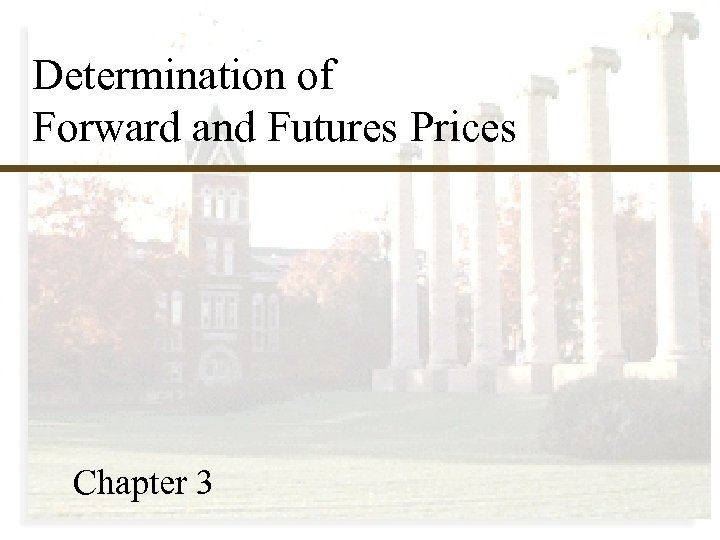Determination of Forward and Futures Prices Chapter 3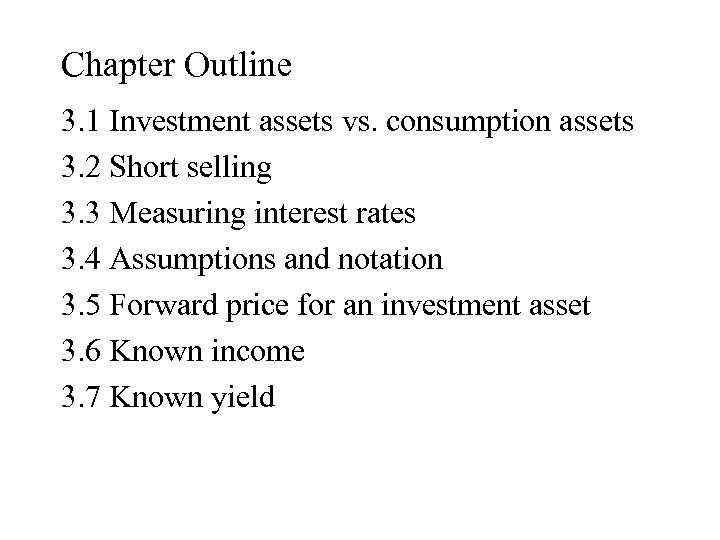Chapter Outline 3. 1 Investment assets vs. consumption assets 3. 2 Short selling 3. 3 Measuring interest rates 3. 4 Assumptions and notation 3. 5 Forward price for an investment asset 3. 6 Known income 3. 7 Known yield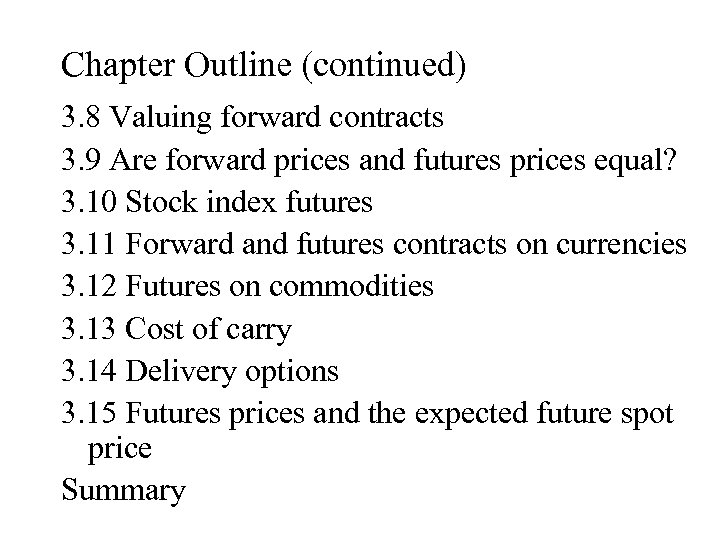Chapter Outline (continued) 3. 8 Valuing forward contracts 3. 9 Are forward prices and futures prices equal? 3. 10 Stock index futures 3. 11 Forward and futures contracts on currencies 3. 12 Futures on commodities 3. 13 Cost of carry 3. 14 Delivery options 3. 15 Futures prices and the expected future spot price Summary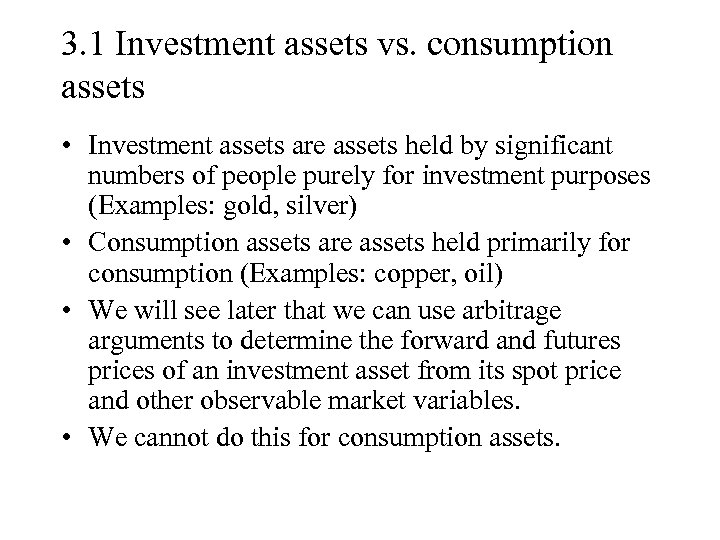3. 1 Investment assets vs. consumption assets • Investment assets are assets held by significant numbers of people purely for investment purposes (Examples: gold, silver) • Consumption assets are assets held primarily for consumption (Examples: copper, oil) • We will see later that we can use arbitrage arguments to determine the forward and futures prices of an investment asset from its spot price and other observable market variables. • We cannot do this for consumption assets.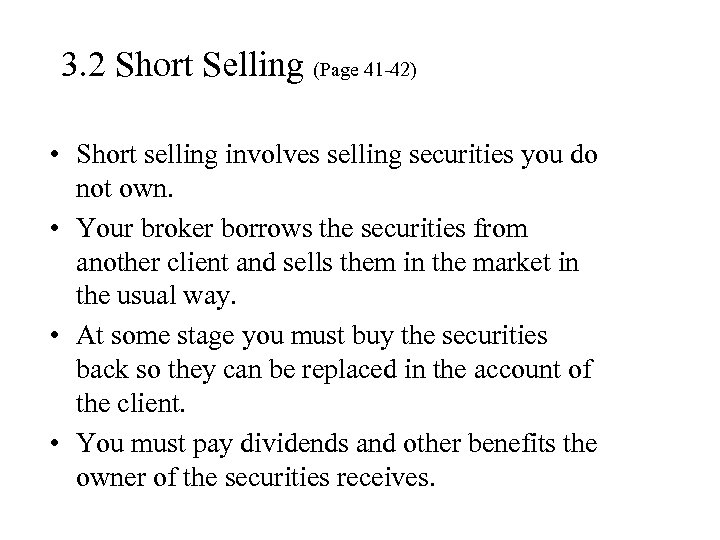3. 2 Short Selling (Page 41 -42) • Short selling involves selling securities you do not own. • Your broker borrows the securities from another client and sells them in the market in the usual way. • At some stage you must buy the securities back so they can be replaced in the account of the client. • You must pay dividends and other benefits the owner of the securities receives.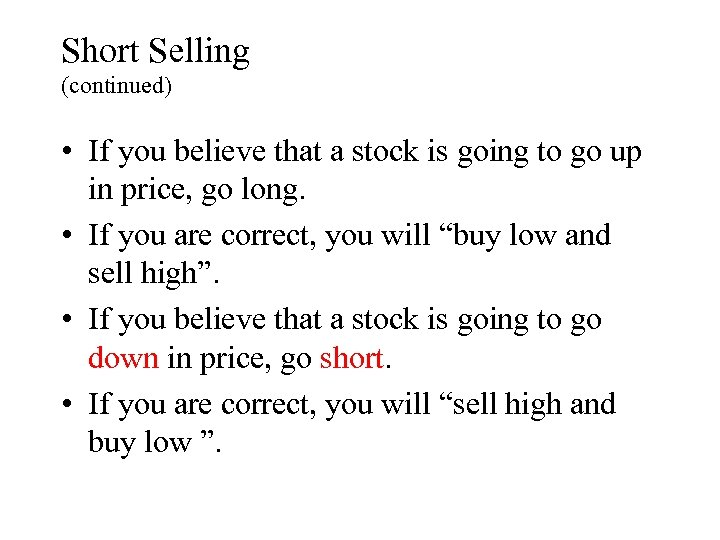Short Selling (continued) • If you believe that a stock is going to go up in price, go long. • If you are correct, you will “buy low and sell high”. • If you believe that a stock is going to go down in price, go short. • If you are correct, you will “sell high and buy low ”.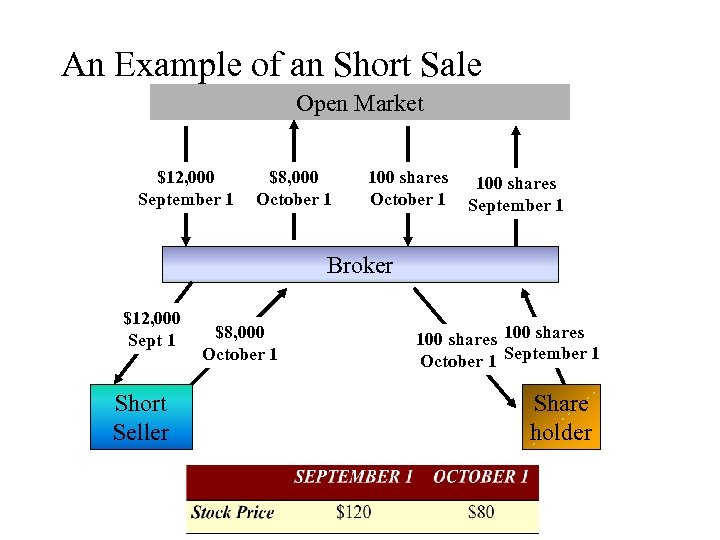An Example of an Short Sale Open Market \$12, 000 September 1 \$8, 000 October 1 100 shares September 1 Broker \$12, 000 Sept 1 Short Seller \$8, 000 October 1 100 shares October 1 September 1 Share holder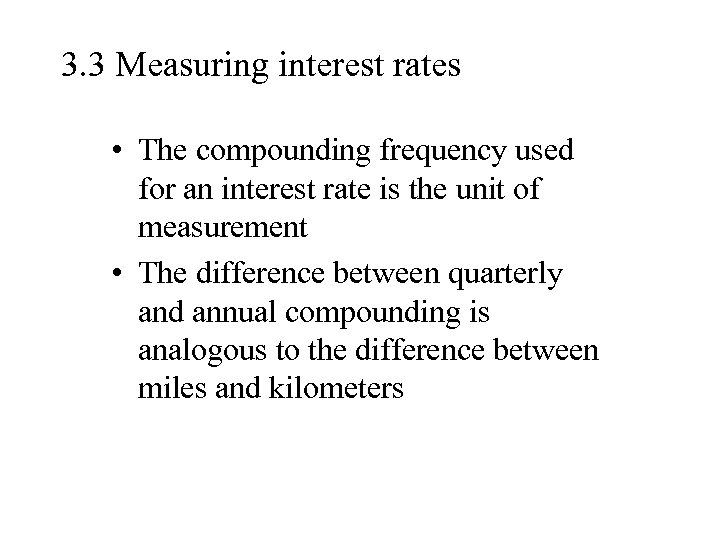3. 3 Measuring interest rates • The compounding frequency used for an interest rate is the unit of measurement • The difference between quarterly and annual compounding is analogous to the difference between miles and kilometersContinuous Compounding (Page 43) • In the limit as we compound more and more frequently we obtain continuously compounded interest rates • \$100 grows to \$100 e. RT when invested at a continuously compounded rate R for time T • \$100 received at time T discounts to \$100 e-RT at time zero when the continuously compounded discount rate is R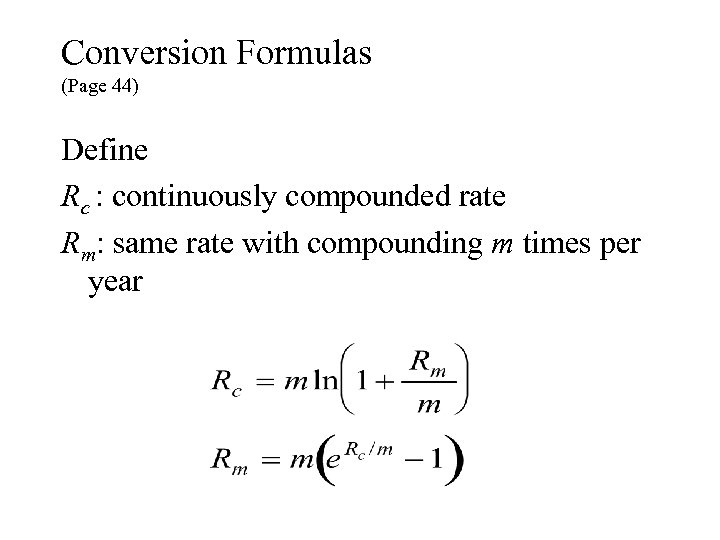Conversion Formulas (Page 44) Define Rc : continuously compounded rate Rm: same rate with compounding m times per year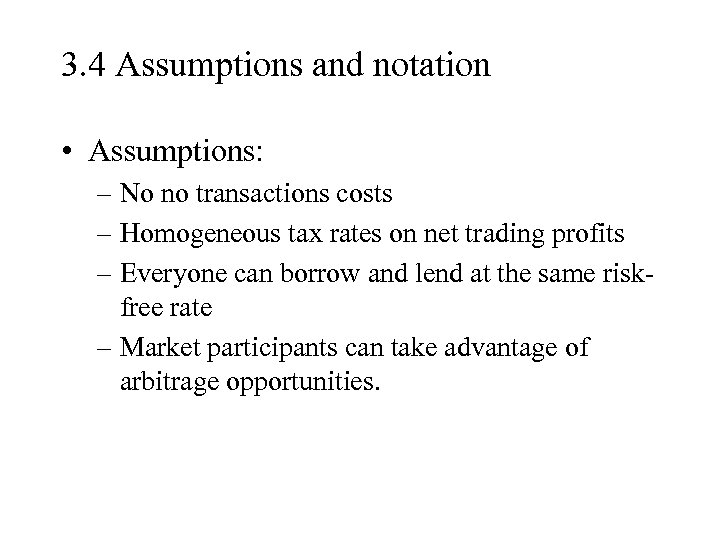3. 4 Assumptions and notation • Assumptions: – No no transactions costs – Homogeneous tax rates on net trading profits – Everyone can borrow and lend at the same riskfree rate – Market participants can take advantage of arbitrage opportunities.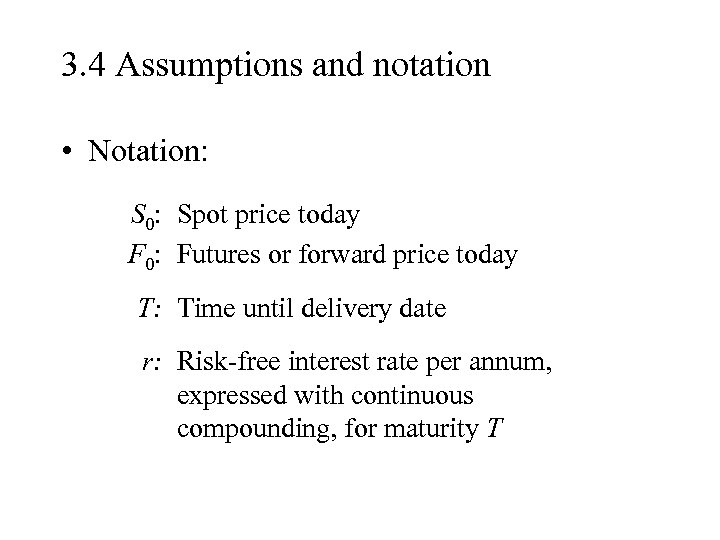3. 4 Assumptions and notation • Notation: S 0: Spot price today F 0: Futures or forward price today T: Time until delivery date r: Risk-free interest rate per annum, expressed with continuous compounding, for maturity T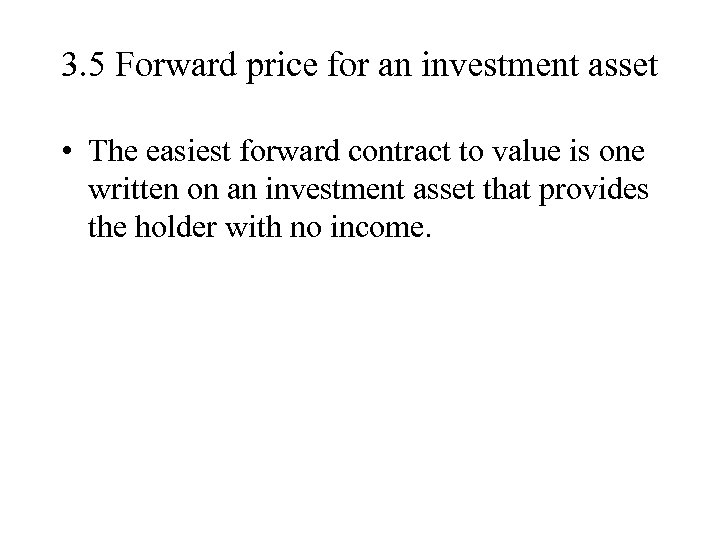3. 5 Forward price for an investment asset • The easiest forward contract to value is one written on an investment asset that provides the holder with no income.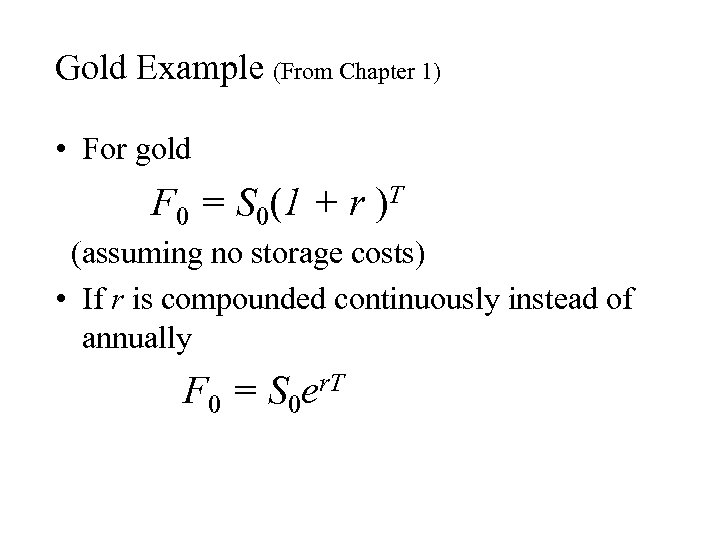Gold Example (From Chapter 1) • For gold F 0 = S 0(1 + r )T (assuming no storage costs) • If r is compounded continuously instead of annually F 0 = S 0 er. T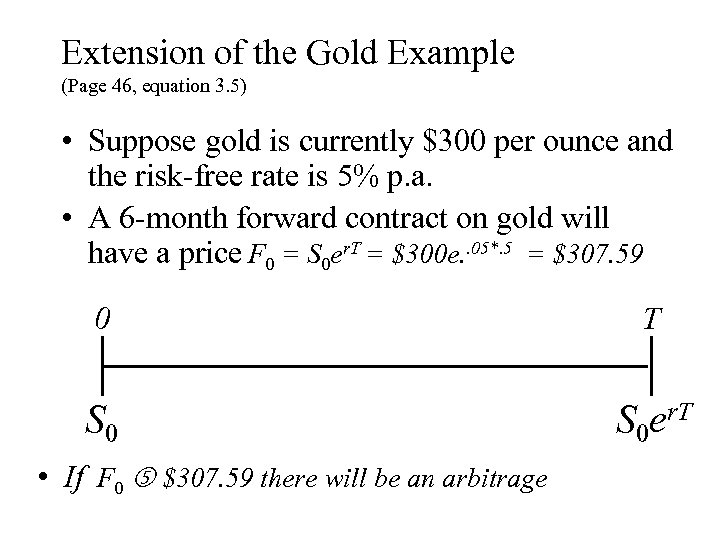Extension of the Gold Example (Page 46, equation 3. 5) • Suppose gold is currently \$300 per ounce and the risk-free rate is 5% p. a. • A 6 -month forward contract on gold will have a price F 0 = S 0 er. T = \$300 e. . 05*. 5 = \$307. 59 0 T S 0 er. T • If F 0 \$307. 59 there will be an arbitrage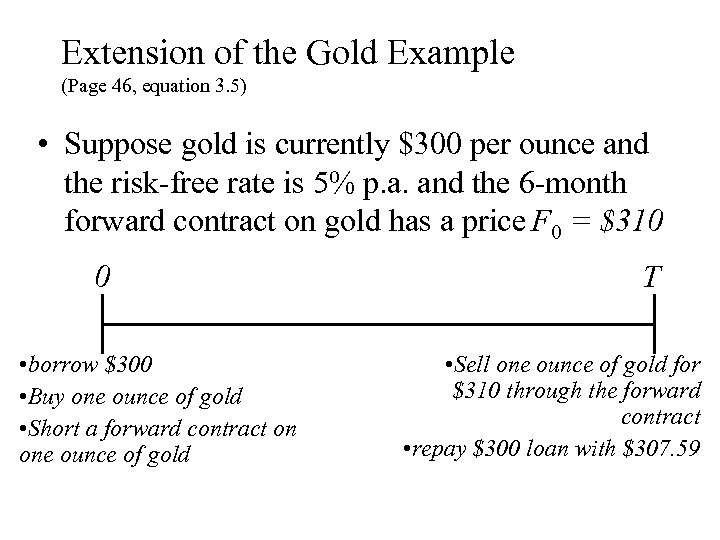Extension of the Gold Example (Page 46, equation 3. 5) • Suppose gold is currently \$300 per ounce and the risk-free rate is 5% p. a. and the 6 -month forward contract on gold has a price F 0 = \$310 0 • borrow \$300 • Buy one ounce of gold • Short a forward contract on one ounce of gold T • Sell one ounce of gold for \$310 through the forward contract • repay \$300 loan with \$307. 59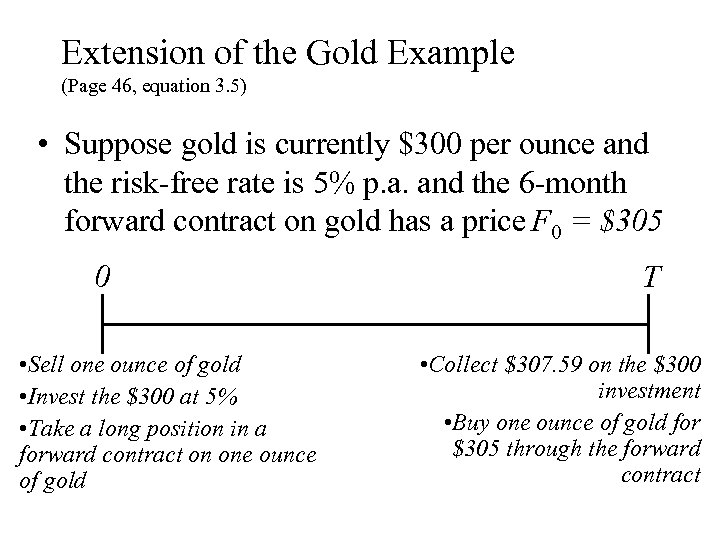Extension of the Gold Example (Page 46, equation 3. 5) • Suppose gold is currently \$300 per ounce and the risk-free rate is 5% p. a. and the 6 -month forward contract on gold has a price F 0 = \$305 0 • Sell one ounce of gold • Invest the \$300 at 5% • Take a long position in a forward contract on one ounce of gold T • Collect \$307. 59 on the \$300 investment • Buy one ounce of gold for \$305 through the forward contract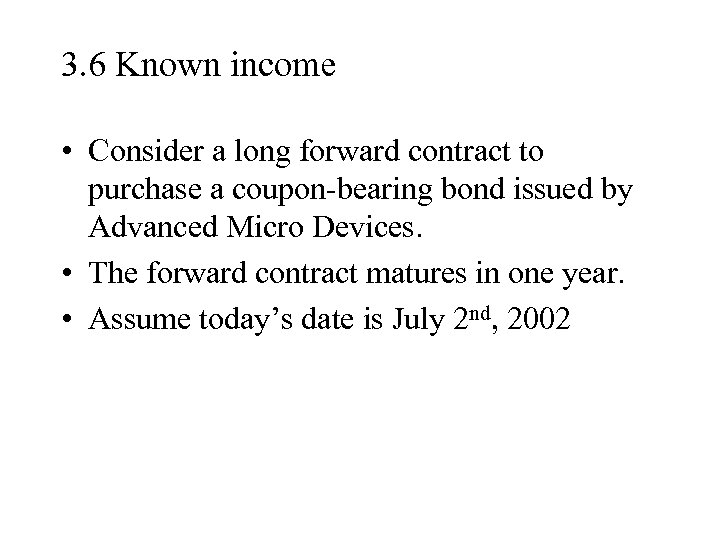3. 6 Known income • Consider a long forward contract to purchase a coupon-bearing bond issued by Advanced Micro Devices. • The forward contract matures in one year. • Assume today’s date is July 2 nd, 2002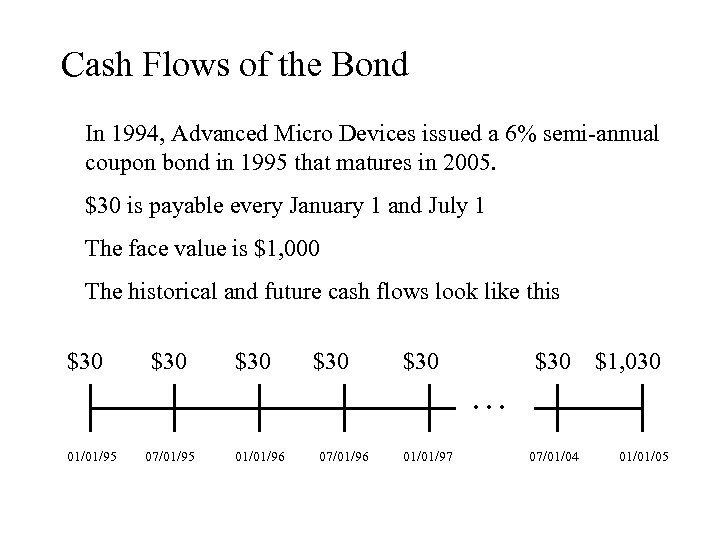Cash Flows of the Bond In 1994, Advanced Micro Devices issued a 6% semi-annual coupon bond in 1995 that matures in 2005. \$30 is payable every January 1 and July 1 The face value is \$1, 000 The historical and future cash flows look like this \$30 01/01/95 \$30 07/01/95 \$30 01/01/96 \$30 07/01/96 \$30 01/01/97 … \$30 07/01/04 \$1, 030 01/01/05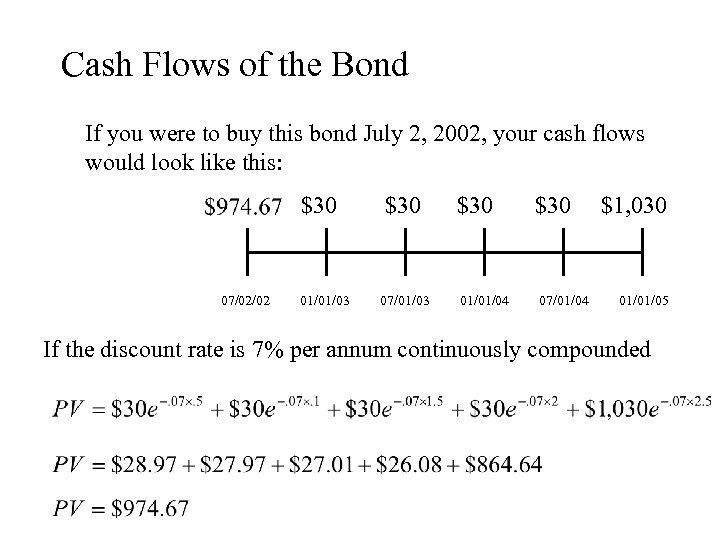Cash Flows of the Bond If you were to buy this bond July 2, 2002, your cash flows would look like this: \$30 07/02/02 01/01/03 \$30 07/01/03 \$30 01/01/04 \$30 07/01/04 \$1, 030 01/01/05 If the discount rate is 7% per annum continuously compounded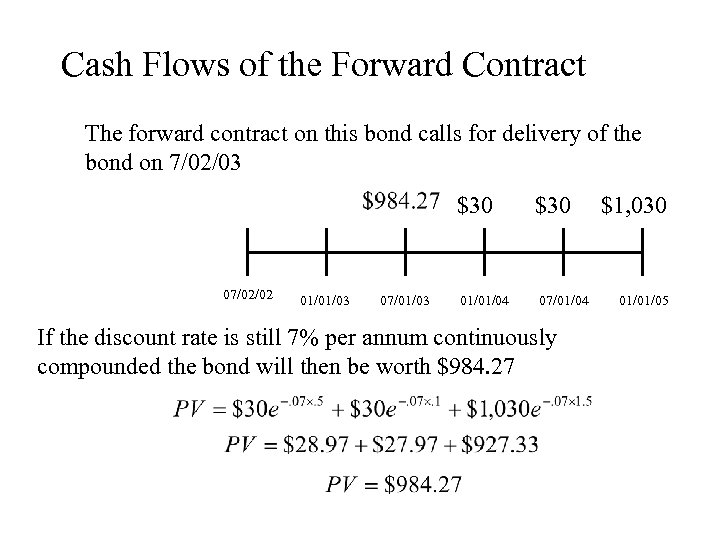Cash Flows of the Forward Contract The forward contract on this bond calls for delivery of the bond on 7/02/03 \$30 07/02/02 01/01/03 07/01/03 01/01/04 \$30 07/01/04 If the discount rate is still 7% per annum continuously compounded the bond will then be worth \$984. 27 \$1, 030 01/01/05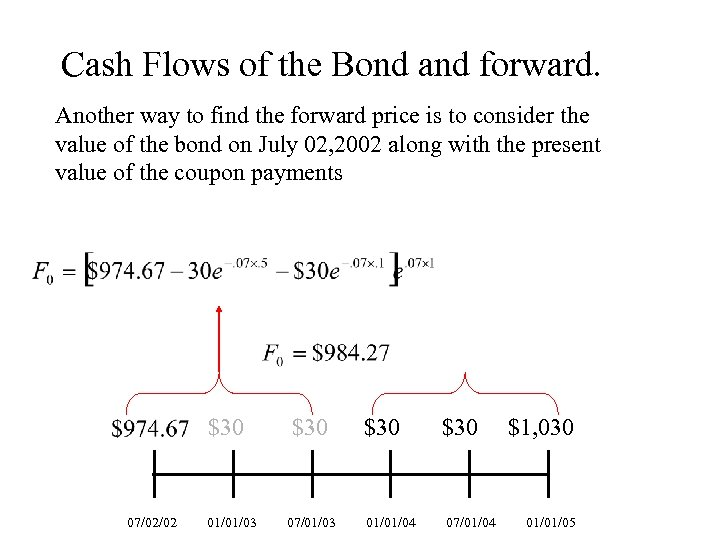Cash Flows of the Bond and forward. Another way to find the forward price is to consider the value of the bond on July 02, 2002 along with the present value of the coupon payments \$30 07/02/02 01/01/03 \$30 07/01/03 \$30 01/01/04 \$30 07/01/04 \$1, 030 01/01/05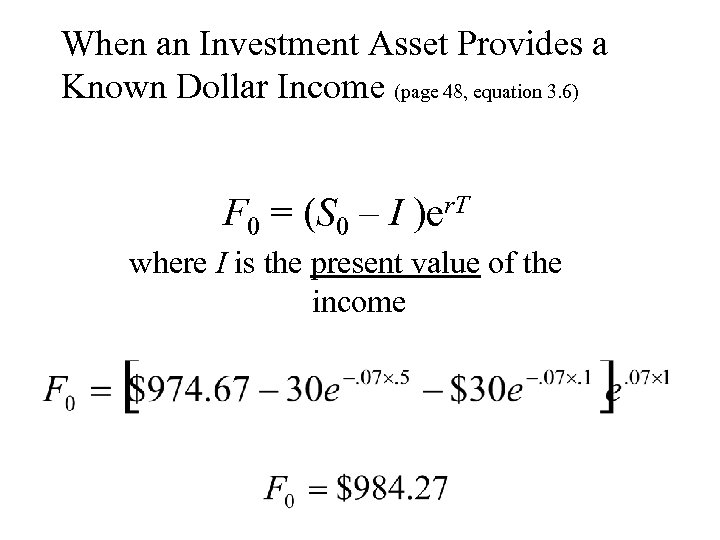When an Investment Asset Provides a Known Dollar Income (page 48, equation 3. 6) F 0 = (S 0 – I )er. T where I is the present value of the income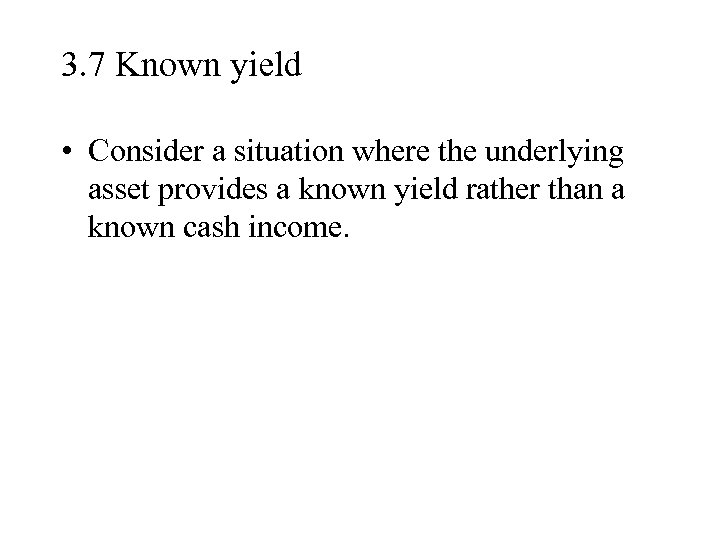3. 7 Known yield • Consider a situation where the underlying asset provides a known yield rather than a known cash income.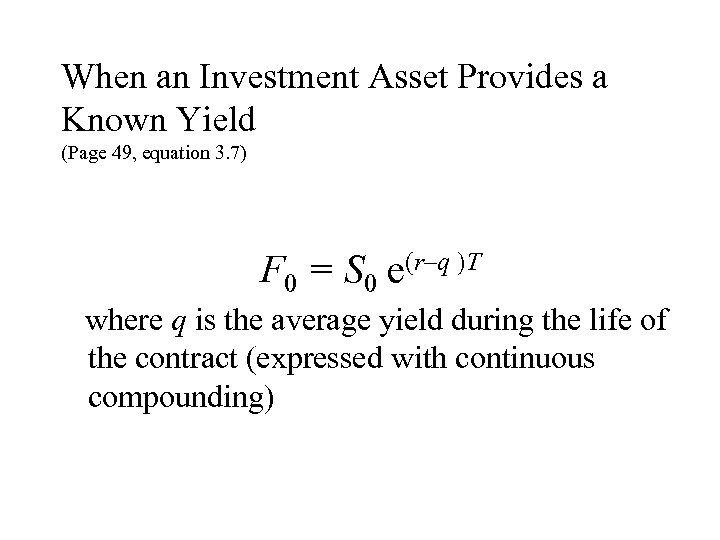When an Investment Asset Provides a Known Yield (Page 49, equation 3. 7) F 0 = S 0 e(r–q )T where q is the average yield during the life of the contract (expressed with continuous compounding)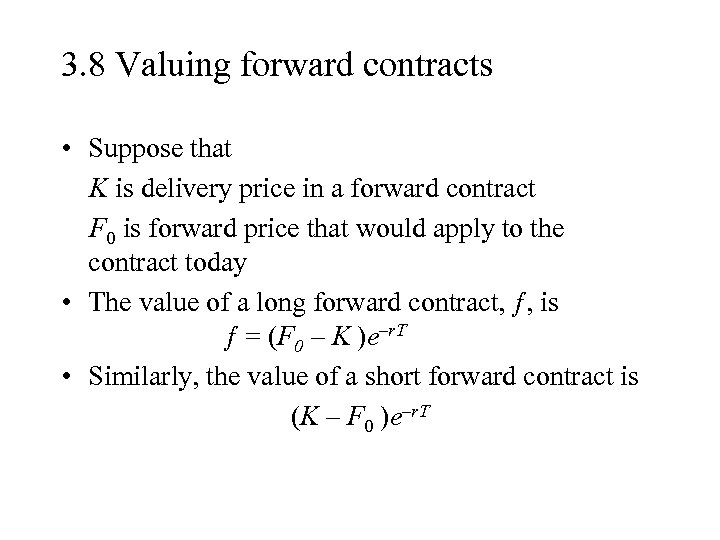3. 8 Valuing forward contracts • Suppose that K is delivery price in a forward contract F 0 is forward price that would apply to the contract today • The value of a long forward contract, ƒ, is ƒ = (F 0 – K )e–r. T • Similarly, the value of a short forward contract is (K – F 0 )e–r. T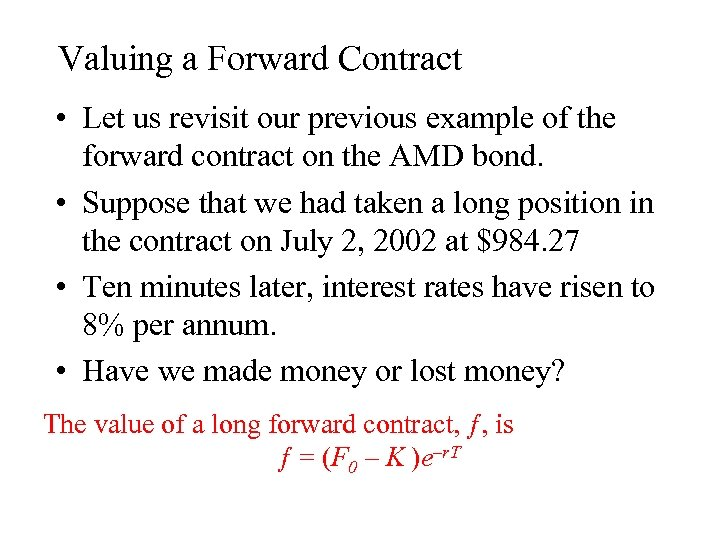Valuing a Forward Contract • Let us revisit our previous example of the forward contract on the AMD bond. • Suppose that we had taken a long position in the contract on July 2, 2002 at \$984. 27 • Ten minutes later, interest rates have risen to 8% per annum. • Have we made money or lost money? The value of a long forward contract, ƒ, is ƒ = (F 0 – K )e–r. T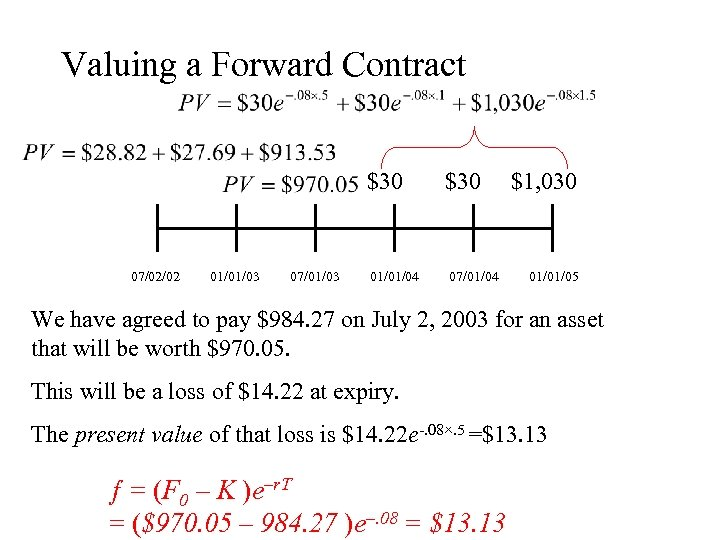Valuing a Forward Contract \$30 07/02/02 01/01/03 07/01/03 01/01/04 \$30 07/01/04 \$1, 030 01/01/05 We have agreed to pay \$984. 27 on July 2, 2003 for an asset that will be worth \$970. 05. This will be a loss of \$14. 22 at expiry. The present value of that loss is \$14. 22 e-. 08×. 5 =\$13. 13 ƒ = (F 0 – K )e–r. T = (\$970. 05 – 984. 27 )e–. 08 = \$13. 13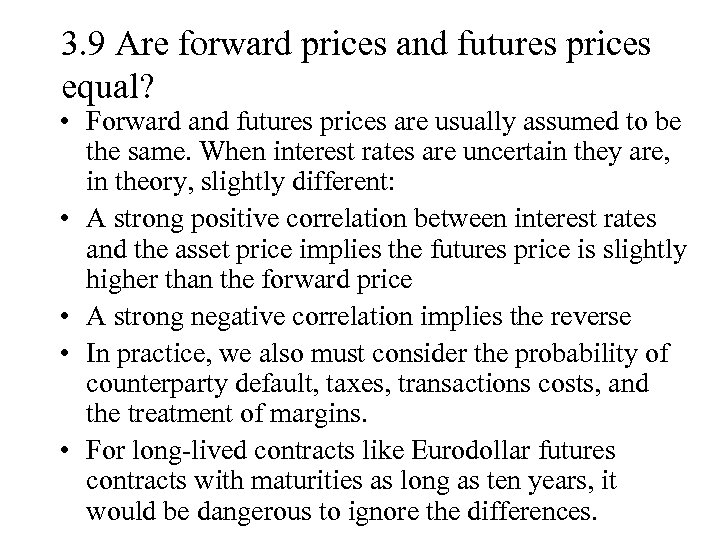3. 9 Are forward prices and futures prices equal? • Forward and futures prices are usually assumed to be the same. When interest rates are uncertain they are, in theory, slightly different: • A strong positive correlation between interest rates and the asset price implies the futures price is slightly higher than the forward price • A strong negative correlation implies the reverse • In practice, we also must consider the probability of counterparty default, taxes, transactions costs, and the treatment of margins. • For long-lived contracts like Eurodollar futures contracts with maturities as long as ten years, it would be dangerous to ignore the differences.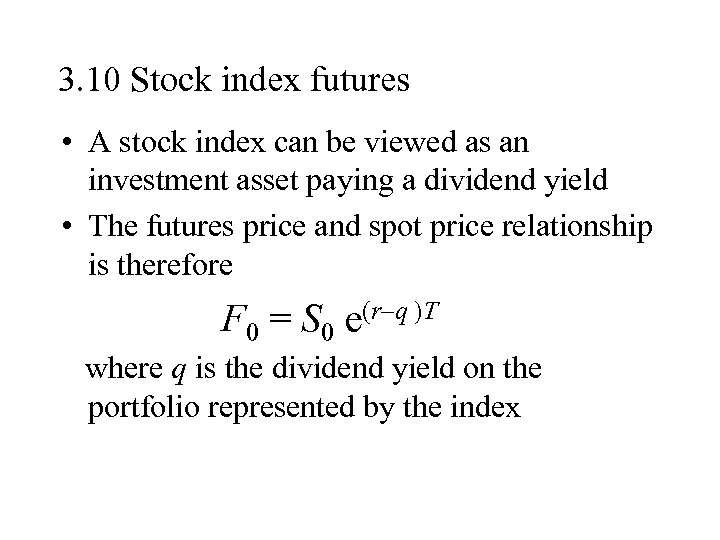3. 10 Stock index futures • A stock index can be viewed as an investment asset paying a dividend yield • The futures price and spot price relationship is therefore F 0 = S 0 e(r–q )T where q is the dividend yield on the portfolio represented by the index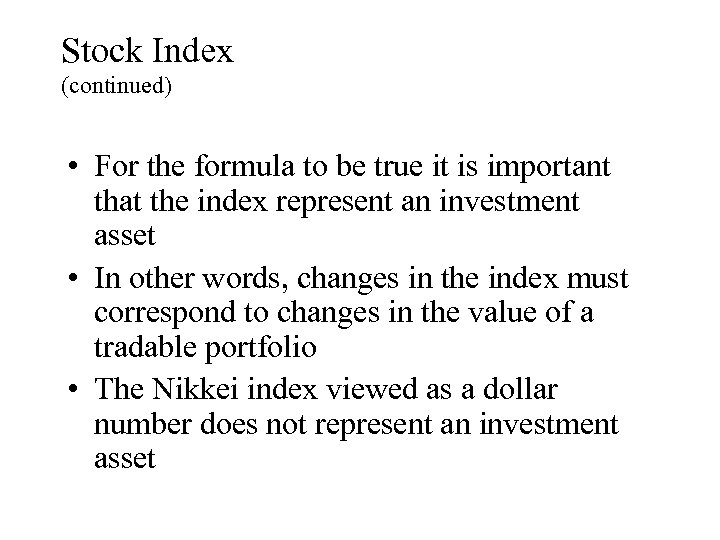Stock Index (continued) • For the formula to be true it is important that the index represent an investment asset • In other words, changes in the index must correspond to changes in the value of a tradable portfolio • The Nikkei index viewed as a dollar number does not represent an investment asset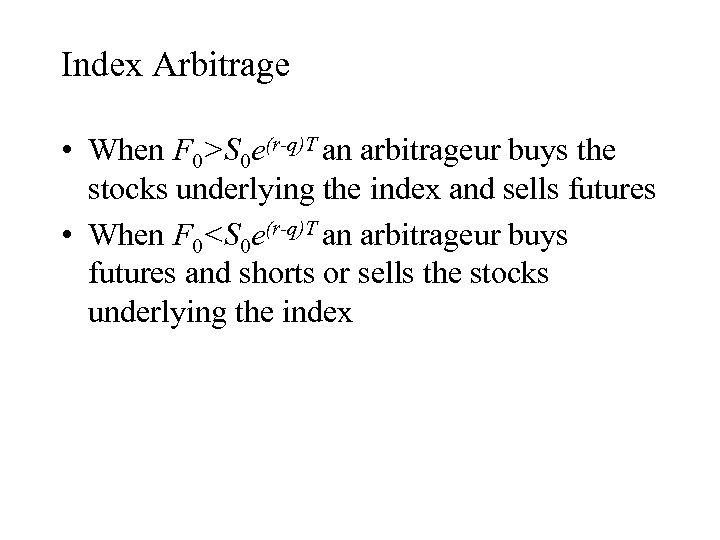Index Arbitrage • When F 0>S 0 e(r-q)T an arbitrageur buys the stocks underlying the index and sells futures • When F 0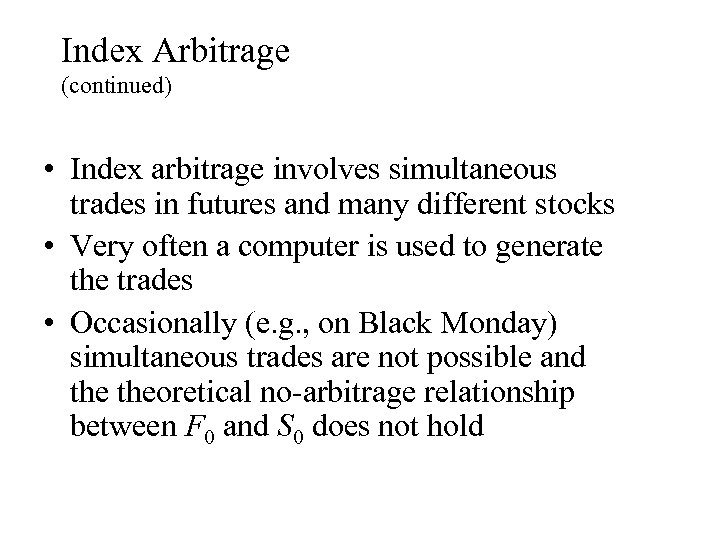Index Arbitrage (continued) • Index arbitrage involves simultaneous trades in futures and many different stocks • Very often a computer is used to generate the trades • Occasionally (e. g. , on Black Monday) simultaneous trades are not possible and theoretical no-arbitrage relationship between F 0 and S 0 does not hold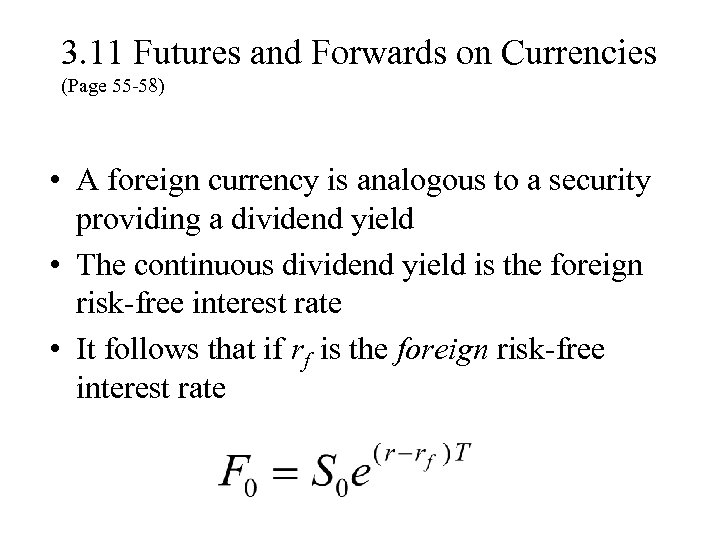3. 11 Futures and Forwards on Currencies (Page 55 -58) • A foreign currency is analogous to a security providing a dividend yield • The continuous dividend yield is the foreign risk-free interest rate • It follows that if rf is the foreign risk-free interest rate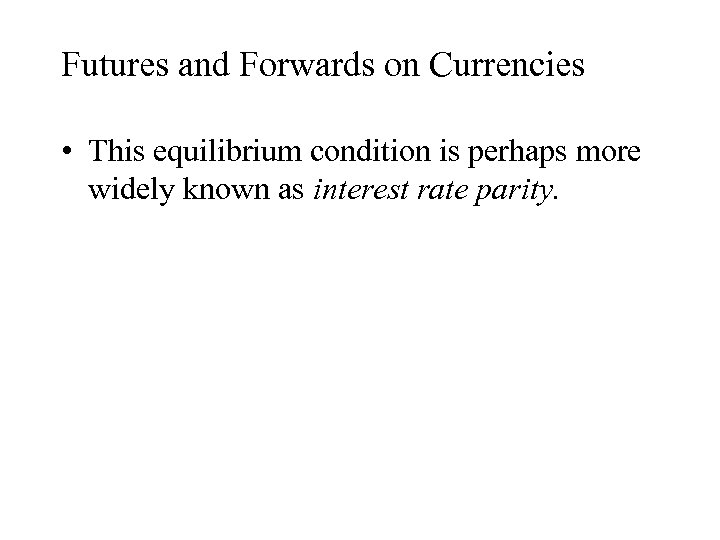Futures and Forwards on Currencies • This equilibrium condition is perhaps more widely known as interest rate parity.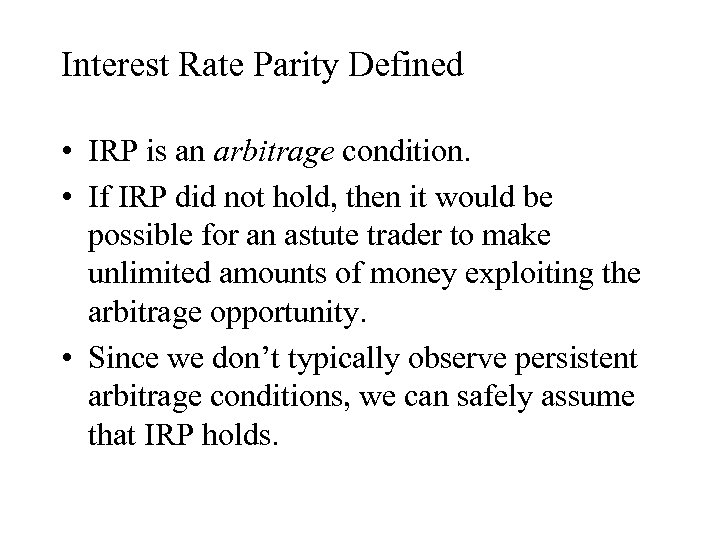Interest Rate Parity Defined • IRP is an arbitrage condition. • If IRP did not hold, then it would be possible for an astute trader to make unlimited amounts of money exploiting the arbitrage opportunity. • Since we don’t typically observe persistent arbitrage conditions, we can safely assume that IRP holds.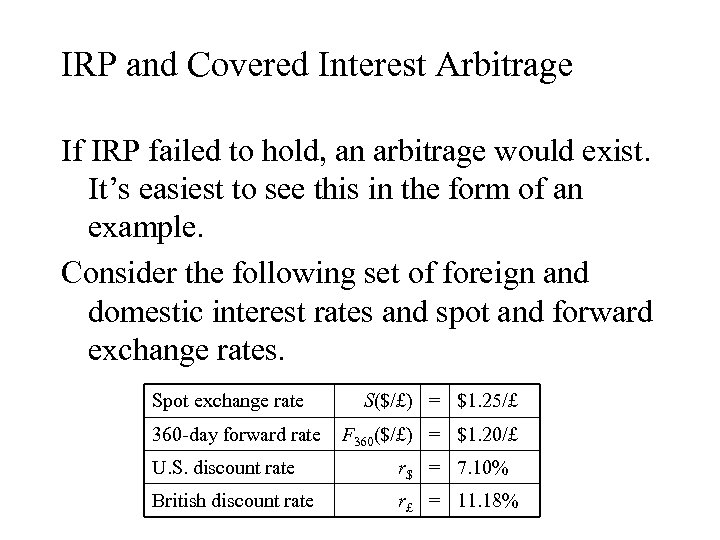IRP and Covered Interest Arbitrage If IRP failed to hold, an arbitrage would exist. It’s easiest to see this in the form of an example. Consider the following set of foreign and domestic interest rates and spot and forward exchange rates. Spot exchange rate 360 -day forward rate S(\$/£) = \$1. 25/£ F 360(\$/£) = \$1. 20/£ U. S. discount rate r\$ = 7. 10% British discount rate r£ = 11. 18%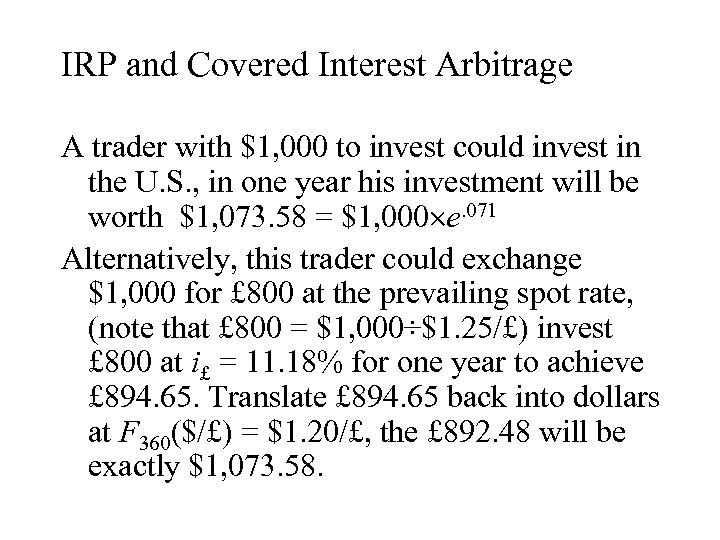IRP and Covered Interest Arbitrage A trader with \$1, 000 to invest could invest in the U. S. , in one year his investment will be worth \$1, 073. 58 = \$1, 000 e. 071 Alternatively, this trader could exchange \$1, 000 for £ 800 at the prevailing spot rate, (note that £ 800 = \$1, 000÷\$1. 25/£) invest £ 800 at i£ = 11. 18% for one year to achieve £ 894. 65. Translate £ 894. 65 back into dollars at F 360(\$/£) = \$1. 20/£, the £ 892. 48 will be exactly \$1, 073. 58.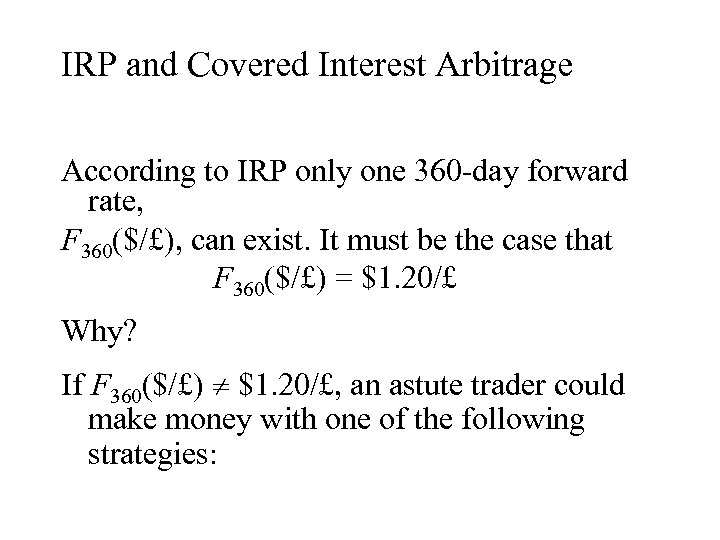IRP and Covered Interest Arbitrage According to IRP only one 360 -day forward rate, F 360(\$/£), can exist. It must be the case that F 360(\$/£) = \$1. 20/£ Why? If F 360(\$/£) \$1. 20/£, an astute trader could make money with one of the following strategies: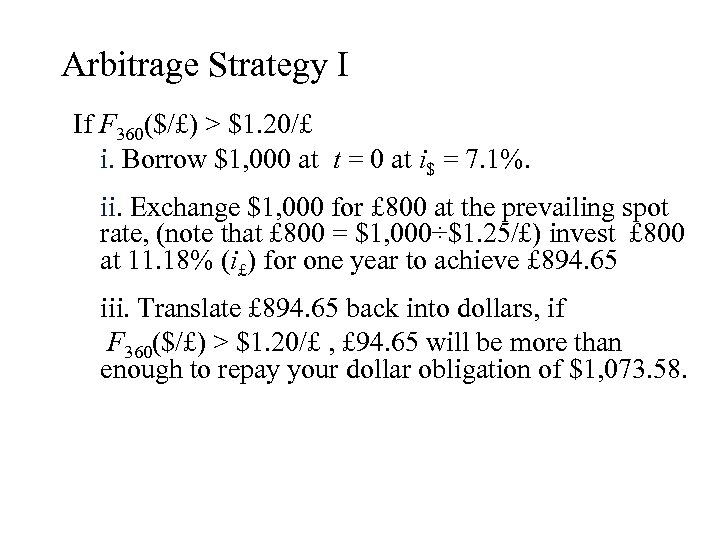Arbitrage Strategy I If F 360(\$/£) > \$1. 20/£ i. Borrow \$1, 000 at t = 0 at i\$ = 7. 1%. ii. Exchange \$1, 000 for £ 800 at the prevailing spot rate, (note that £ 800 = \$1, 000÷\$1. 25/£) invest £ 800 at 11. 18% (i£) for one year to achieve £ 894. 65 iii. Translate £ 894. 65 back into dollars, if F 360(\$/£) > \$1. 20/£ , £ 94. 65 will be more than enough to repay your dollar obligation of \$1, 073. 58.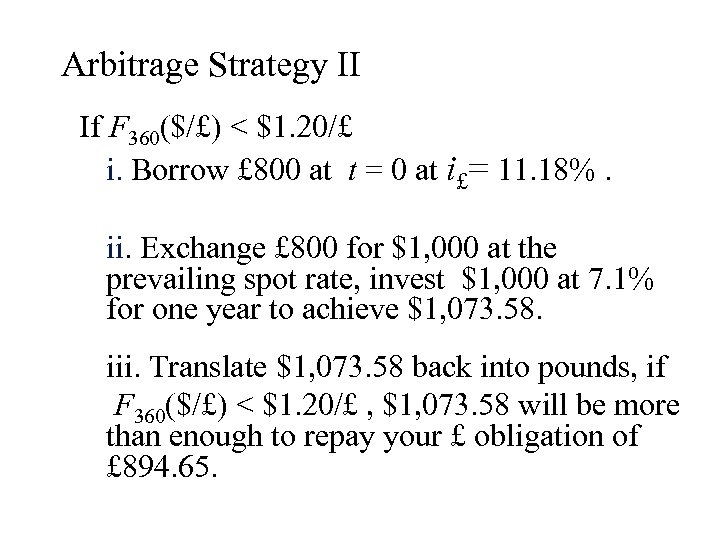Arbitrage Strategy II If F 360(\$/£) < \$1. 20/£ i. Borrow £ 800 at t = 0 at i£= 11. 18%. ii. Exchange £ 800 for \$1, 000 at the prevailing spot rate, invest \$1, 000 at 7. 1% for one year to achieve \$1, 073. 58. iii. Translate \$1, 073. 58 back into pounds, if F 360(\$/£) < \$1. 20/£ , \$1, 073. 58 will be more than enough to repay your £ obligation of £ 894. 65.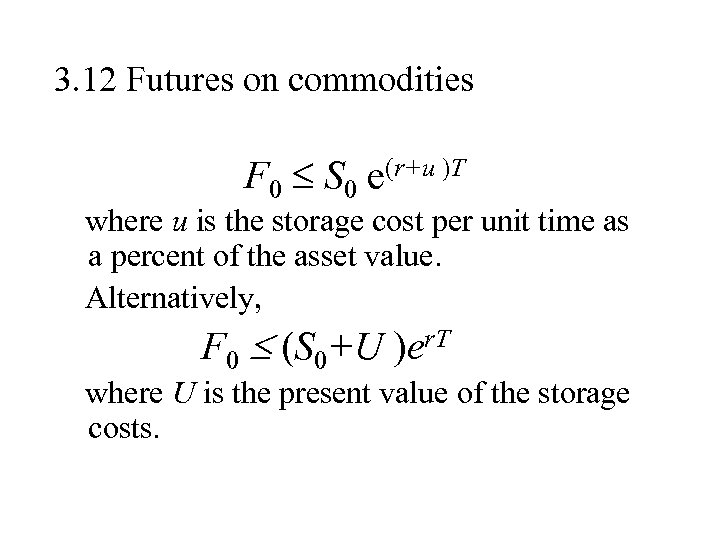3. 12 Futures on commodities F 0 S 0 e(r+u )T where u is the storage cost per unit time as a percent of the asset value. Alternatively, F 0 (S 0+U )er. T where U is the present value of the storage costs.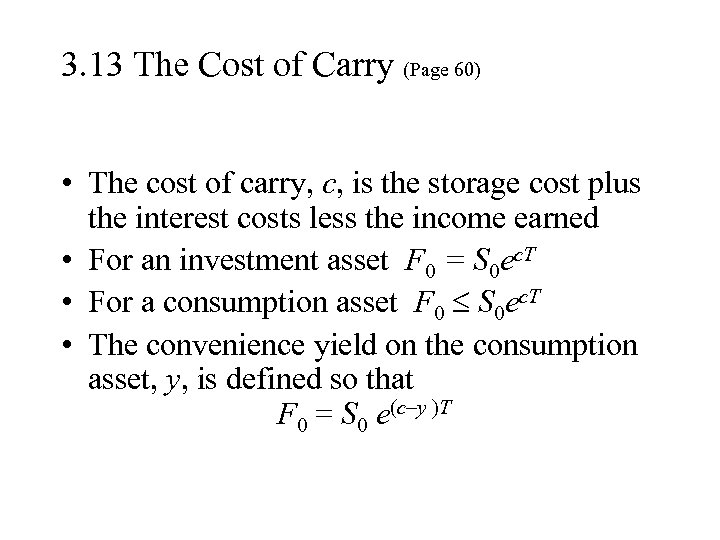3. 13 The Cost of Carry (Page 60) • The cost of carry, c, is the storage cost plus the interest costs less the income earned • For an investment asset F 0 = S 0 ec. T • For a consumption asset F 0 S 0 ec. T • The convenience yield on the consumption asset, y, is defined so that F 0 = S 0 e(c–y )T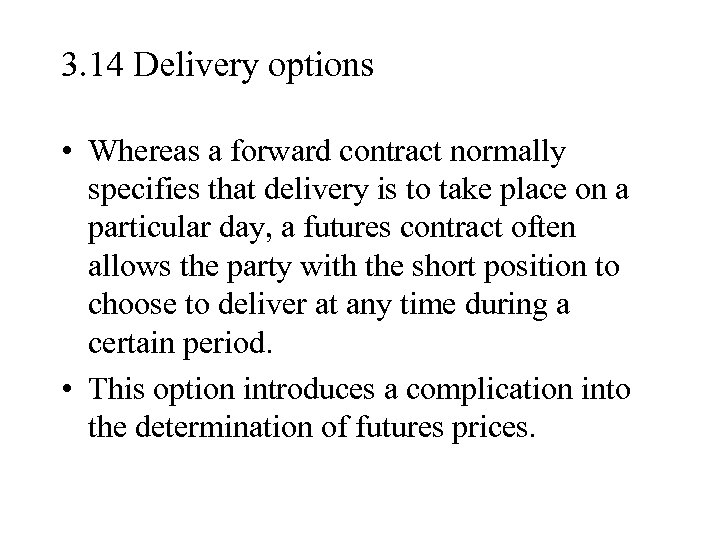3. 14 Delivery options • Whereas a forward contract normally specifies that delivery is to take place on a particular day, a futures contract often allows the party with the short position to choose to deliver at any time during a certain period. • This option introduces a complication into the determination of futures prices.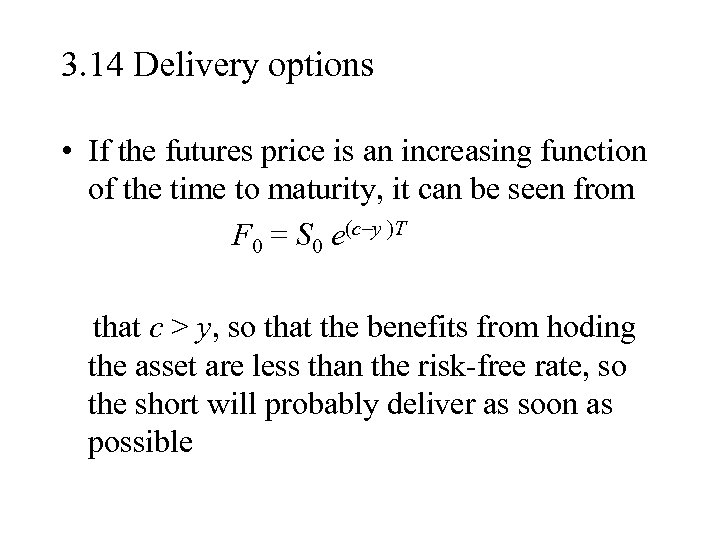3. 14 Delivery options • If the futures price is an increasing function of the time to maturity, it can be seen from F 0 = S 0 e(c–y )T that c > y, so that the benefits from hoding the asset are less than the risk-free rate, so the short will probably deliver as soon as possible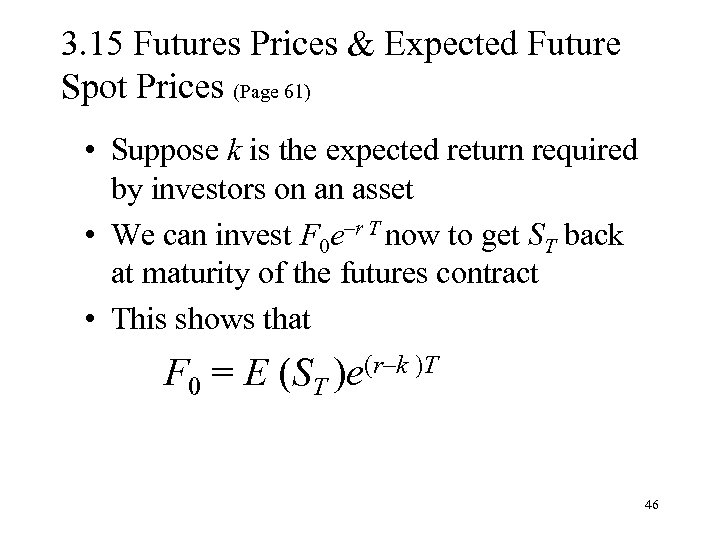3. 15 Futures Prices & Expected Future Spot Prices (Page 61) • Suppose k is the expected return required by investors on an asset • We can invest F 0 e–r T now to get ST back at maturity of the futures contract • This shows that F 0 = E (ST )e(r–k )T 46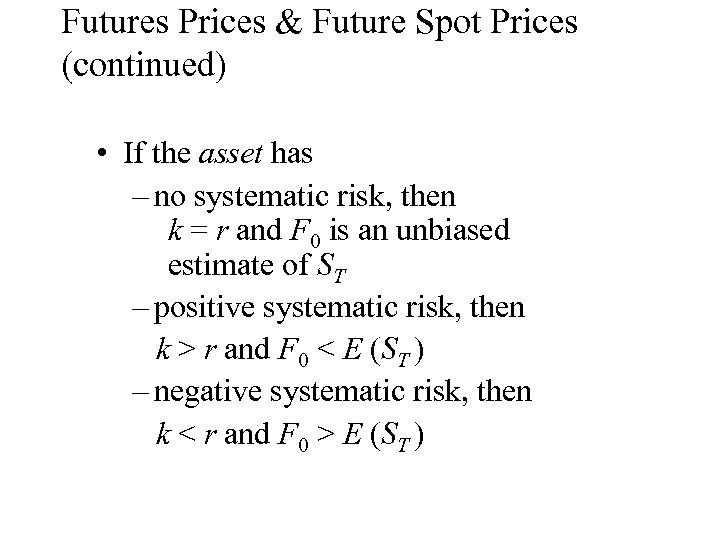Futures Prices & Future Spot Prices (continued) • If the asset has – no systematic risk, then k = r and F 0 is an unbiased estimate of ST – positive systematic risk, then k > r and F 0 < E (ST ) – negative systematic risk, then k < r and F 0 > E (ST )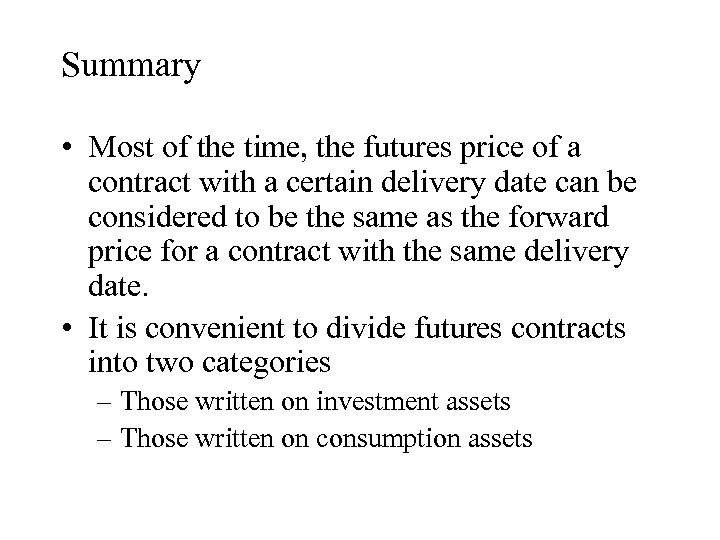Summary • Most of the time, the futures price of a contract with a certain delivery date can be considered to be the same as the forward price for a contract with the same delivery date. • It is convenient to divide futures contracts into two categories – Those written on investment assets – Those written on consumption assets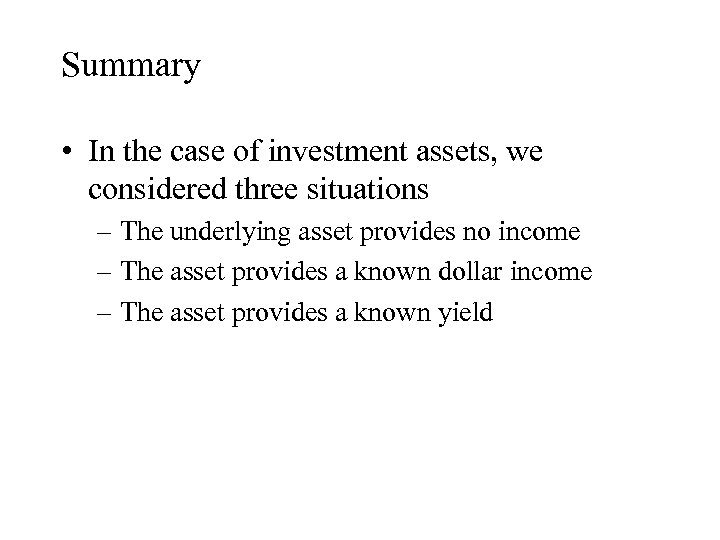Summary • In the case of investment assets, we considered three situations – The underlying asset provides no income – The asset provides a known dollar income – The asset provides a known yield